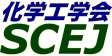## \$B9V1i%W%m%0%i%`!J%;%C%7%g%sJL!K(B

### \$B%(%l%/%H%m%K%/%9(B

#### M213-M215

\$B:G=*99?7F|;~!'(B2007-03-18 23:33:23

2006\$BG/(B12\$B7n(B2\$BF|!A(B24\$BF|\$K7G:\\$5\$l\$F\$\$\$?2>%W%m%0%i%`\$H\$O2q>lL>\$,JQ99\$5\$l\$F\$\$\$^\$9\$N\$G\$4Cm0U\$/\$@\$5\$\$!#(B

\$B9V1i(B
\$B;~9o(B
\$B9V1i(B
\$BHV9f(B
\$B9V1iBjL\!?H/I=\$B%-!<%o!<%I(B\$BJ,N`(B
\$BHV9f(B
\$B\$BHV9f(B
M\$B2q>l(B \$BBh(B2\$BF|(B
(13:00\$B!A(B14:00)\$B!!(B(\$B:BD9(B \$B6aF#(B \$BOBIW(B)
13:00\$B!A(B 13:20M213\$BH>F3BN%W%m%;%9@_7W\$N\$?\$a\$NNL;RJ,;RF0NO3XK!\$K4p\$E\$/%^%k%A%U%#%8%C%/%9%7%_%e%l!<%?\$N3+H/(B
(\$BElKLBg1!9)(B/JST\$B\$5\$-\$,\$1(B) \$B!{(B(\$B@5(B)\$B5WJ](B \$BI4;J(B\$B!&(B(\$BElKLBg1!9)(B) \$BDZ0f(B \$B=(9T(B\$B!&(B(\$B@5(B)\$B8E;3(B \$BDL5W(B\$B!&(B\$BH+;3(B \$BK>(B\$B!&(B(\$B@5(B)\$B1sF#(B \$BL@(B\$B!&(B(\$B@5(B)\$B9b1)(B \$BMN=<(B\$B!&(BDel Carpio Carlos A.\$B!&(B(\$BElKLBgL\$Mh%;(B/\$BElKLBg1!9)(B) (\$B@5(B)\$B5\K\(B \$BL@(B
quantum chemical molecular dynamics
semiconductor fabrication process
multi-physics simulation
11-a43
13:20\$B!A(B 13:40M214\$BEE>l2<\$K\$*\$1\$k6d%\$%*%s\$N3H;6(B
(\$B5~Bg(B) \$B!{(B(\$B@5(B)\$B2.Ln(B \$BJ84](B
forced diffusion of ion
migration of silver
electrochemical engineering
11-b72
13:40\$B!A(B 14:00M215\$BH>F3BN%Q%C%1!<%8\$N\$O\$s\$@%j%U%m!<6/EYM=B,5;=Q(B-\$B\$=\$N(B 2-
(\$B=;M'%Y!<%/%i%\$%H(B) \$B!{>BED(B \$B9'(B\$B!&(B\$BEDCf(B \$B9(G7(B\$B!&(B(\$B5~Bg1!9)(B) \$B_@ED(B \$B3X(B\$B!&(B\$BCSED(B \$BE0(B
packaging material
moisture sensitivity level
computer simulation
11-d562

\$B9V1i%W%m%0%i%`(B
\$B2=3X9)3X2q(B \$BBh(B72\$BG/2q(B

(C) 2007 (\$B
Most recent update: 2007-03-18 23:33:23
For more information contact \$B2=3X9)3X2q4X@>;YIt(B \$BBh(B72\$BG/2q(B \$BLd\$\$9g\$;78(B
E-mail: inquiry-72awww2.scej.org
This page was generated byeasp 2.22; proghtml 2.20b (C)1999-2006 kawase